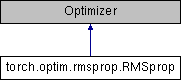Caffe2 - Python API A deep learning, cross platform ML framework
torch.optim.rmsprop.RMSprop Class Reference
Inheritance diagram for torch.optim.rmsprop.RMSprop:## Public Member Functions

def __init__ (self, params, lr=1e-2, alpha=0.99, eps=1e-8, weight_decay=0, momentum=0, centered=False)

def __setstate__ (self, state)

def step (self, closure=None)

## Detailed Description

```Implements RMSprop algorithm.

Proposed by G. Hinton in his
`course <http://www.cs.toronto.edu/~tijmen/csc321/slides/lecture_slides_lec6.pdf>`_.

The centered version first appears in `Generating Sequences
With Recurrent Neural Networks <https://arxiv.org/pdf/1308.0850v5.pdf>`_.

Arguments:
params (iterable): iterable of parameters to optimize or dicts defining
parameter groups
lr (float, optional): learning rate (default: 1e-2)
momentum (float, optional): momentum factor (default: 0)
alpha (float, optional): smoothing constant (default: 0.99)
eps (float, optional): term added to the denominator to improve
numerical stability (default: 1e-8)
centered (bool, optional) : if ``True``, compute the centered RMSProp,
the gradient is normalized by an estimation of its variance
weight_decay (float, optional): weight decay (L2 penalty) (default: 0)```

Definition at line 5 of file rmsprop.py.

## Member Function Documentation

 def torch.optim.rmsprop.RMSprop.step ( self, closure = `None` )
```Performs a single optimization step.

Arguments:
closure (callable, optional): A closure that reevaluates the model
and returns the loss.
```

Definition at line 49 of file rmsprop.py.

The documentation for this class was generated from the following file: